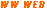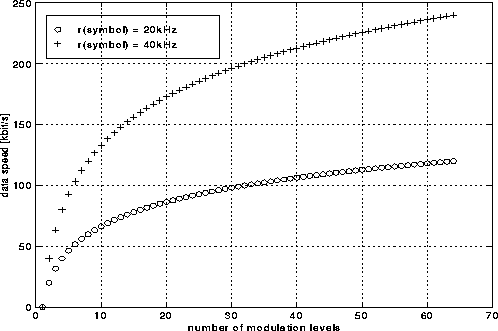### JPL's Wireless Communication Reference Website

#### Chapter: Analog and Digital Transmission Section: Spread Spectrum , Frequency Hopping# WISSCE: A Hybrid DS/FH CDMA Communication System

Contributed by Jack Glas, Lucent.

WISSCE (Wireless Indoor Spread Spectrum Communication Equipment) is an ad-hoc (nomadic) communication system meant to operate in the 2.4 GHz ISM-band providing random/multi-access wireless communication links. It is an example of a system exploiting the hybrid Direct Sequence / Frequency Hopping spread spectrum technique. This page covers the design considerations that are related to the this CDMA technique. WISSCEwas a research project at T.U. Delft in The Netherlands.

Frequency regulation and WISSCE's full-duplex operation result in a total available bandwidth of 10 MHz, a number that can be considered as a boundary condition. Choices that will be motivated in this section include: selecting the hopping rate (relative to the symbol rate), the pn-code and FH sequence length (NDS and NFH) and choosing a modulation format.

## Data speed

It is obvious that the user desires a high transmission capacity (overall data speed). However, an high data speed also leads to conflicts with user demands like low purchasing costs'', short access time'', meeting legal requirements'' and others. As a result a compromise should be found.

The relation between occupied bandwidth, spreading factor and data speed can be written as:where Gp is the direct sequence spreading factor. dMOD expresses the extra bandwidth usage due to the applied modulation type which is usually small in comparison with the other bandwidth term. A plot illustrating this trade-off is shown in figure 1.The bit-rate (rd)can be expressed in terms of the symbol-rate (rsymb):From these formulas follows that the data speed can be increased independently of the occupied bandwidth by increasing the number of modulation levels (= number of bits per symbol). However, increasing this number of bits per symbol also increases both the implementation cost and the bit error probability.

Summarizing: increasing the data speed leads to either a larger bandwidth occupancy or higher hardware costs and a worse bit error rate.

Figure 2 shows the trade-off between data speed and the number of modulation levels. This plot makes clear that it is advantageous to have a rather small number of modulation levels: the data speed increases logarithmic with the number of levels while the data detection complexity increases linearly. Appropriate choices are 8, 16 or 32.## CDMA technique

Secondly we focus on the trade-offs related to the hybrid DS/FH CDMA technique . Fast Frequency Hopping not only increases the cost of the frequency synthesizer, it also complicates data detection algorithm as an hop'' introduces a transient response that the data detector has to cope with. For this reasons we propose an hybrid system approach in which the hop rate'' is equal to the symbol rate''. Every data-symbol is spread with a complete pn-code while successive symbols are transmitted in different FH-channels as illustrated in figure 3. The actual spreading gain in such a system is equal to pn-code sequence length, while Frequency Hopping only provides protection against the Near-Far effect.Following this concept, a user-address consists of a frequency-hopping pattern of length NFH and NFH (possibly different) pn-codes of length NDS. The start of a new set of pn-codes and an FH-sequence are linked. This is important when regarding the code-synchronization problem. An example user-address existing of an FH-sequence of length 7 and 7 pn-codes is shown in figure 3. Every data-symbol is combined with a pn-code causing the direct-sequence spreading. Subsequent data-symbols are transmitted in different FH-channels according to a certain sequence to perform FH-spreading. As a result we use neither fast nor slow frequency-hopping. A frequency-synthesizer only has to hop'' at a speed equal to the symbol-rate.

For the relation between NDS, NFH, the symbol-rate and the occupied bandwidth holds:For constant BWtotal/rsymb and a small dMOD, the trade-off curves shown in figure 4 can be obtained for three values of BWtotal/rsymb. Concerning this trade-off the following remarks can be made:

• It is likely that the DS-code will be made using shift-registers (so-called Linear Feedback Shift Register sequences [Gol67]). The code-length is then 2n-1 with n being an integer. For this reason, only the marked points in figure 4 are possible trade-off points.
• Increasing NDS is advantageous (increasing of spreading gain) and can be realized at low implementation costs.
• Increasing NFH results in the requirement for a more expensive FH-frequency-synthesizer and is for this reason undesired. A minimum number of FH-channels is however required to limit the influence of the near-far effect (near-interference).

To find a minimum value for NFH, user demands like reliable operation'' and large user capacity'' are translated into a technical compromise that maximally 2 near users may be present. As the frequency-hopping sequences are chosen in such a way that they have at most 2 partial hits with another sequence [Gla96, p.63] [MT92], this compromise translates into the worst case possibility of having at most 4 partial hits per FH-sequence. Furthermore we need at least two frequency-hopping channels without near-interference to allow proper synchronization [Gla96, p.86]. As a result the minimum value of NFH is 6, a vertical line representing this value is also shown in figure 4.

After evaluating other trade-offs as well we will address the actual choice of the values of NDS and NFH.

## Modulation format

An issue not yet addressed is the choice of modulation format. Requirements towards this digital modulation format are low implementation costs, the possibility of applying multi-level modulation (having multiple bits per symbol) and a low susceptibility to effects caused by the indoor radio-path. Three likely candidates for the modulation format exist: amplitude modulation, phase modulation and frequency modulation. Detection of amplitude modulated symbols is problematic in combination with multi-level modulation while frequency-hopping in combination with phase shift keying is susceptible to multi-path effects and requires phase-continuous frequency hopping or a return-to-zero code. This leaves frequency shift keying (FSK) as the better alternative.

A well known disadvantage of FSK is that it requires a larger bandwidth (higher value of dMOD) however this value will still be small in comparison with the already occupied spread spectrum bandwidth. Increasing the number of modulation levels is possible by using more than two frequencies. The resulting modulation technique is referred to as multiple frequency shift keying (MFSK). An additional property of frequency modulation is the possibility of non-coherent data detection. This saves hardware costs as a carrier tracking loop is not required if frequency errors are small.

## Fixing the system parameters

We will now address the actual parameter choices for WISSCE.

To obtain a communication system which is competitive in data speed with currently available (wire line) modems, we select the gross bit-rate to be 80 kbit/s at a BER of 10-3. Depending on the application (and the required BER) this will lead to different data speeds after error correction.

To find the symbol-rate the number of bits per symbol has to be determined. A large number of bits per symbol leads to a more complex data detection circuit while it decreases the required bandwidth. By choosing the number of bits per symbol to be 4, the modulation format becomes 16-MFSK and the symbol-speed 20 ksymbol/s.

Evaluating the equation for the total bandwidth now results in a spreading factor of about 500. This number has to be shared between NDS (length of DS-code) and NFH according to the formula that relates the total bandwidth to these parameters. Increasing NDS is advantageous as it increases the spreading gain while increasing NFH leads to a higher reduction of the near-far effect. This trade-off was shown in figure 4.

Two constraints exist: the minimum value of NFH is 6 and for NDS only certain numbers are possible (2n-1). From earlier discussions followed that NDS should be as high as possible while increasing NFH leads to higher implementation costs. From figure 4 we conclude that a proper value for NDS is 63, for the resulting value of NFH follows 7 and the chip-rate (rc) is 1260 kHz (symbol rate times NFH).

The power density spectrum of a DS-spread signal has a sync2 shape where the zero-values are the chip-rate apart. For this reason the FH-spacing is fixed to be equal to the chip-rate (DFH = 1260 kHz). To obtain orthogonal frequency shift keying, the frequency-distance between the MFSK-frequencies should be an integer times the symbol-rate. To minimize dMOD, DFSK is fixed to be 20 kHz.

### References

Gla96
Jack P.F. Glas.
Non-Cellular Wireless Communication Systems.
PhD thesis, Delft University of Technology, December 1996.
ISBN: 90-5326-024-2.Gol67
S. W. Golomb.
Shift Register Sequences.
Holden-Day Inc., San Francisco, California, 1967.

MT92
Svetislav V. Maric and Edward L. Titlebaum.
A class of frequency hop codes with nearly ideal characteristics for use in multiple-access a spread-spectrum communications and radar and sonar systems.
IEEE Transactions on Communications, COM-40(9):1442-1447, September 1992.

WIS
WISSCE-team, Circuits and Systems group. WISSCE home-page.• BER of a DS/FH CDMA System employing MFSK Modulation